HOME plateWON | World!OfNumbers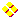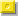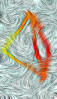Details ofPalindromic TetrahedralsPalindromic Tetrahedrals

Legend
```
F_ba  Decomposition of the basenumber in its prime factors.
F_pt  Decomposition of the palindromic tetrahedral.
Date  Date of discovery by the author.Hypertext links to related topics in number theory

```

Factorizations
```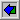F_ba  336 =
2  x  2  x  2  x  2  x  3  x  7
F_pt  6378736 =
2  x  2  x  2  x  2  x  7  x  13  x  13  x  337F_ba  21 =
3  x  7
F_pt  1771 =
7  x  11  x  23F_ba  17 =
PRIME
F_pt  969 =
3  x  17  x  19F_ba  2 =
PRIME
F_pt  4 =
2  x  2F_ba  1 =
1
F_pt  1 =
1F_ba  0 =
0
F_pt  0 =
0

```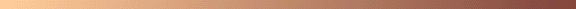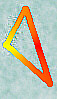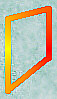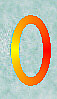[TOP OF PAGE]

Patrick De Geest - Belgium- Short Bio - Some Pictures